So far we mostly used polygonal meshes to represent shapes. While meshes are the easiest to render and the most versatile, there are other ways to represent shapes in 2d and 3d. One way which is used frequently is signed distance fields. Signed distance fields allow for cheaper raytracing, smoothly letting different shapes flow into each other and saving lower resolution textures for higher quality images.

We’re going to start by generating signed distance fields with functions in 2 dimensions, but later continue by generating and using them in 3d. I’m going to use the worldspace coordinates to make everything as independent from scaling and uv coordinates as possible, so if you’re unsure how that works, look at this tutorial about planar mapping which explains what’s happening.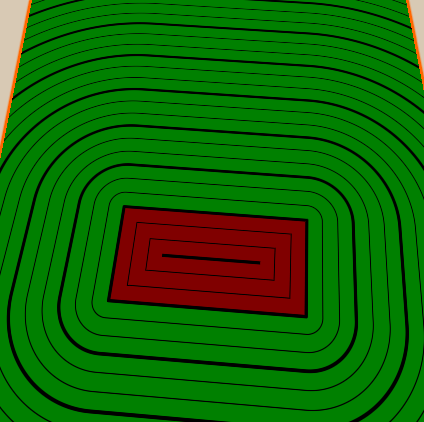## Base Setup

From the base of the planar mapping shader we throw out the properties for now because we’ll do the technical base for now. Then we’ll write the world position to the vertex to fragment struct directly instead of transforming it to the uvs first. As a last point for preparation we’ll write a new function which will calculate the scene and return the distance to the nearest surface. Then we’ll call the function and use the result as the color.

``````Shader "Tutorial/034_2D_SDF_Basics"{
SubShader{
//the material is completely non-transparent and is rendered at the same time as the other opaque geometry
Tags{ "RenderType"="Opaque" "Queue"="Geometry"}

Pass{
CGPROGRAM

#include "UnityCG.cginc"

#pragma vertex vert
#pragma fragment frag

struct appdata{
float4 vertex : POSITION;
};

struct v2f{
float4 position : SV_POSITION;
float4 worldPos : TEXCOORD0;
};

v2f vert(appdata v){
v2f o;
//calculate the position in clip space to render the object
o.position = UnityObjectToClipPos(v.vertex);
//calculate world position of vertex
o.worldPos = mul(unity_ObjectToWorld, v.vertex);
return o;
}

float scene(float2 position) {
// calculate distance to nearest surface
return 0;
}

fixed4 frag(v2f i) : SV_TARGET{
float dist = scene(i.worldPos.xz);
fixed4 col = fixed4(dist, dist, dist, 1);
return col;
}

ENDCG
}
}
FallBack "Standard" //fallback adds a shadow pass so we get shadows on other objects
}
``````

I’ll write all of the functions we write for signed distance fields in their own file, so we can easily reuse them later. For that we create a new file. In it we add include guards by first checking if a preprocessor varible isn’t defined yet, if it isn’t we define it and end the if condition after the functions we want to include. The advantage of adding this is that if we include the file twice (for example if we include two different files which both have functions we want and they both include the same file) it doesn’t break the shader. If you’re sure that’s never going to happen, feel free to not add them.

``````// in include file

// include guards that keep the functions from being included more than once
#ifndef SDF_2D
#define SDF_2D

// functions

#endif
``````

As long as the include file in the same directory as the main shader, we can then simply include it with a pragma statement.

``````// in main shader

#include "2D_SDF.cginc"
``````

With this we just see a black surface on our rendered surface and are ready to display the signed distance on it.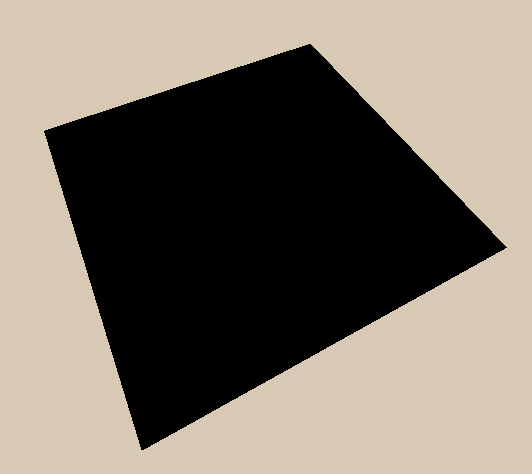## Circle

The simplest signed distance field function is the one for a circle. The function will only take a sample position and a radius of the circle. We start by simply taking the length of the sample position vector. With this we have a point at the (0, 0) position, which is the same as a circle with the radius of 0.

``````float circle(float2 samplePosition, float radius){
return length(samplePosition);
}
``````

We then call the circle function in the scene function and return the distance it returns.

``````float scene(float2 position) {
float sceneDistance = circle(position, 2);
return sceneDistance;
}
``````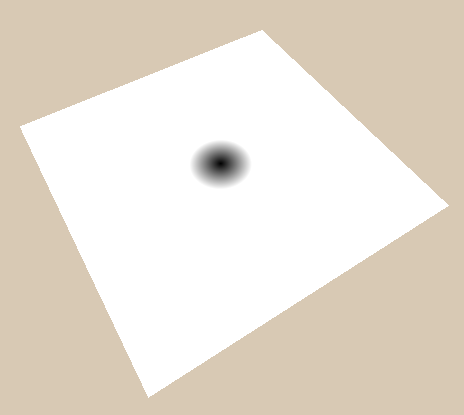Then we include the radius into the calculation. A important thing about signed distance functions is that when inside a object we get the negative distance to the surface (that’s what the “signed” in signed distance field stands for). To grow the circle to the radius we specify we simply subtract the radius from the length. This way the surface, which is everywhere where the function returns 0, moves outward the higher it is. What’s 2 units away from the surface for a circle with the size of 0 is only 1 unit away for a circle with the radius of 1 and is 1 unit inside the circle (value of -1) for a circle with a radius of 3;

``````float circle(float2 samplePosition, float radius){
return length(samplePosition) - radius;
}
``````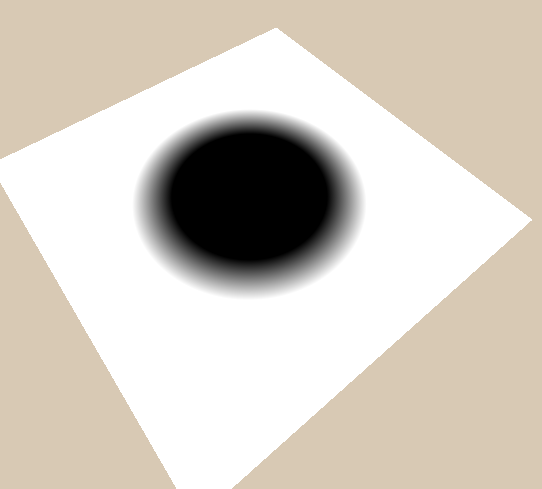Now the only thing we can’t do is to move the circle away from the center. To fix that we can either add a new argument to the circle function to calculate the distance between the sample position and the circle center and subtract the radius from that to define our circle. Or we can redefine the origin by moving the space of the sample point and then get the circle in that space. The later option seems a lot more complex, but because moving things is a operation we want to use on all shapes it’s a lot more flexible and is the way I’m going to explain here.

## Moving

“Transforming the space of a point” sounds a lot more scary than it is. It means that we pass the point into a function and the function changes it so we can still use it afterwards. In the case of translation we simply subtract the offset from the point. The reason we subtract the position when we want to move the shapes in the positive direction is that the shapes we render in a space move in the opposite direction than we move the space into.

For example if we want to draw a sphere at the position `(3, 4)` we have to change the space so `(3, 4)` becomes `(0, 0)` and the operation to do that is to subtract `(3, 4)`. Now if we draw a sphere around the NEW origin it’s at the OLD `(3, 4)` point.

``````// in sdf functions include file

float2 translate(float2 samplePosition, float2 offset){
return samplePosition - offset;
}
``````
``````float scene(float2 position) {
float2 circlePosition = translate(position, float2(3, 2));
float sceneDistance = circle(circlePosition, 2);
return sceneDistance;
}
``````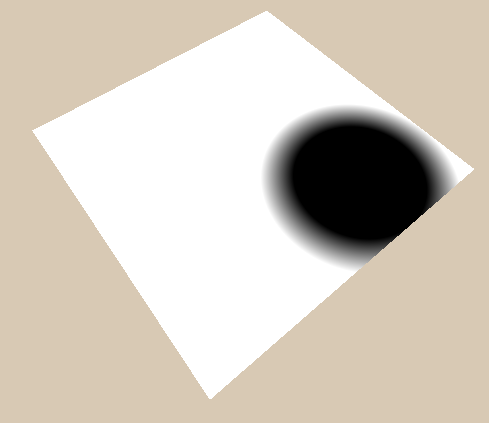## Rectangle

Another simple shape is a rectangle. We start by seeing the components independently. First we get the distance from the center by taking the absolute value. Then, similarly to the circle, we subtract the half size (which is basically like the radius for rectangles). For simply showing how the result looks like we’ll then return one of the components for now.

``````float rectangle(float2 samplePosition, float2 halfSize){
float2 componentWiseEdgeDistance = abs(samplePosition) - halfSize;
return componentWiseEdgeDistance.x;
}
``````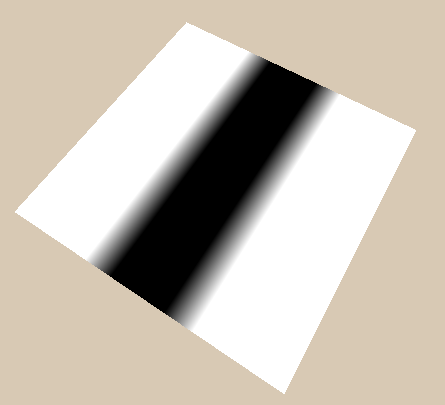We can now get a cheap version of a rectangle by simply returning the bigger component of the 2. This works for many cases, but is wrong because it will not show the correct distance around the corners.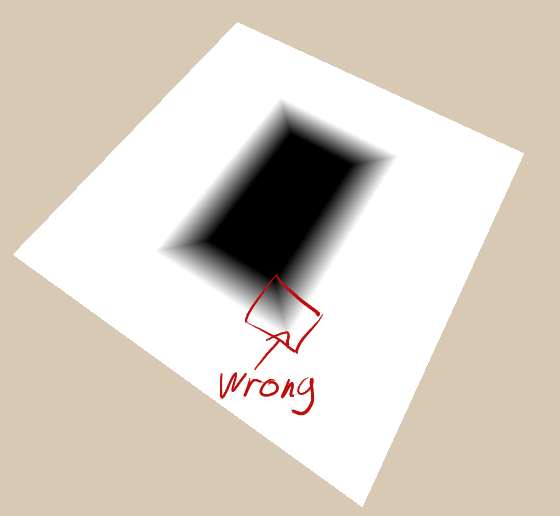We can get the correct values for the rectangle outside of the shape by first taking the maximum between the edge distances and 0 and then taking the length of that.

If we wouldn’t limit the distance to 0 at the lower bound we would just calculate the distance to the corners (where the edgeDistances are `(0, 0)`), but this way the coordinates between the corners don’t go below 0 so it uses the whole edge. The downside of this is that it uses 0 as the distance from the edge for the whole inside of the shape.

The fix to the distance being 0 on the inside of the shape is to generate the inside distance by simply using the formula for the cheap rectangle (taking the maximum value between x and y component) and then ensuring that it’s never above 0 by taking the minimum value between it and 0. Then we can add the outside distance that’s never below 0 and the inside distance that’s never above 0 to get the complete distance function.

``````float rectangle(float2 samplePosition, float2 halfSize){
float2 componentWiseEdgeDistance = abs(samplePosition) - halfSize;
float outsideDistance = length(max(componentWiseEdgeDistance, 0));
float insideDistance = min(max(componentWiseEdgeDistance.x, componentWiseEdgeDistance.y), 0);
return outsideDistance + insideDistance;
}
``````

Because we wrote the translation function in a flexible way previously we can now also use it to move the rectangle whereever we want it’s center to be.

``````float scene(float2 position) {
float2 circlePosition = translate(position, float2(1, 0));
float sceneDistance = rectangle(circlePosition, float2(1, 2));
return sceneDistance;
}
``````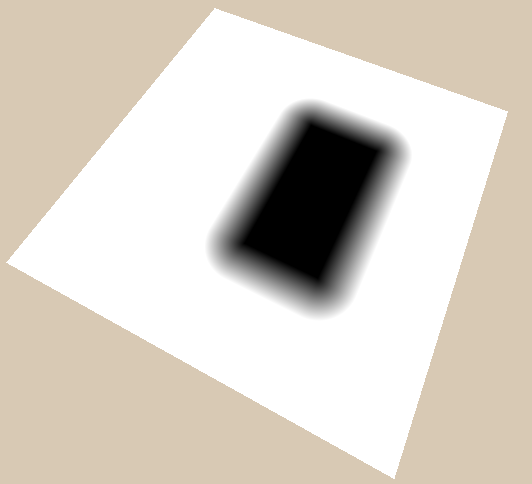## Rotating

Rotating shapes works similarly to moving them. We rotate the coordinate in the opposite direction before calculating the distance to the shape. To make rotations as easy to understand as possible, we multiply the rotation by 2 times pi to get the angle in radians. This way the rotation we pass the function is in rotations, 0.25 is a quarter rotation, 0.5 is half a rotation and 1 is a full rotation (Feel free to convert in another way if that comes more natural to you). Then we also invert it because we have to rotate the position in the inverse direction as the direction we want to rotate the shape in for the same reason we move the position into the negative direction to move the object into the positive direction for the translation.

To calculate the rotated coordinates we first calculate the sine and cosine based on our angle. Hlsl provides the sincos function which calculates both of those values quicker than if we would calculate them independently.

Then we build a new vector, for the x component we use the original x component multiplied by the cosine and the y component multiplied by the sine. We can easily remember this by remembering that the cosine of 0 is 1 and with a rotation of 0 we want the x component of the new vector to be exactly the same as before (multiplying it by 1). The y part that points upwards previously, not contributing anything to the x component of the vector gets rotated to the right, starting at 0 and becoming bigger at first, so that’s exactly the motion a sine describes.

For the y component of the new vector we multiply the cosine with the y component of the old vector and subtract the sine multiplied with the old x comonent. To understand why we subtract here instead of adding the sine multiplied with the x component it’s best to imagine how how a `(1, 0)` vector changes when it rotates clockwise. The y component of the result starts at 0 and then goes down below 0. That’s exactly the opposite behaviour of what a sine does, that’s why we invert it.

``````float2 rotate(float2 samplePosition, float rotation){
const float PI = 3.14159;
float angle = rotation * PI * 2 * -1;
float sine, cosine;
sincos(angle, sine, cosine);
return float2(cosine * samplePosition.x + sine * samplePosition.y, cosine * samplePosition.y - sine * samplePosition.x);
}
``````

Now that we wrote the rotation method we can use it in combination with the translation to make the shape move and rotate.

``````float scene(float2 position) {
float2 circlePosition = position;
circlePosition = rotate(circlePosition, _Time.y);
circlePosition = translate(circlePosition, float2(2, 0));
float sceneDistance = rectangle(circlePosition, float2(1, 2));
return sceneDistance;
}
``````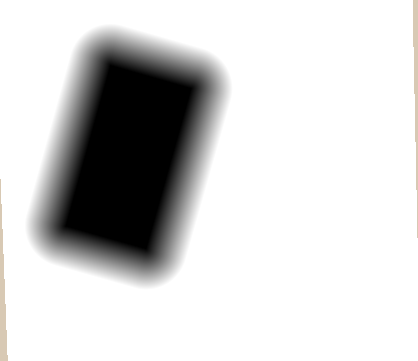In this case we first rotate the object around the center of the whole scene, so the translation is also affected by that rotation. To rotate the shape around it’s own center we first have to move it and then rotate it. With this corrected order we made the center of the shape the center of our coordinate system by the time we rotate it.

``````float scene(float2 position) {
float2 circlePosition = position;
circlePosition = translate(circlePosition, float2(2, 0));
circlePosition = rotate(circlePosition, _Time.y);
float sceneDistance = rectangle(circlePosition, float2(1, 2));
return sceneDistance;
}
``````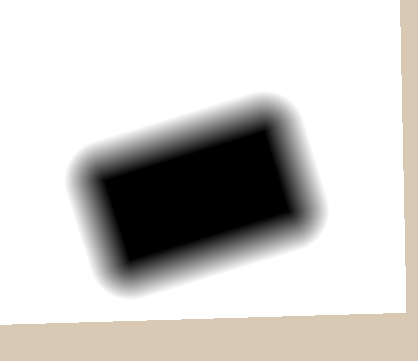## Scaling

Scaling works in a similar way to the other ways of transforming shapes. We divide the coordinates by the scale and by drawing the shape in a scaled down space, they look bigger in the base coordinate system.

``````float2 scale(float2 samplePosition, float scale){
return samplePosition / scale;
}
``````
``````float scene(float2 position) {
float2 circlePosition = position;
circlePosition = translate(circlePosition, float2(0, 0));
circlePosition = rotate(circlePosition, .125);
float pulseScale = 1 + 0.5*sin(_Time.y * 3.14);
circlePosition = scale(circlePosition, pulseScale);
float sceneDistance = rectangle(circlePosition, float2(1, 2));
return sceneDistance;
}
``````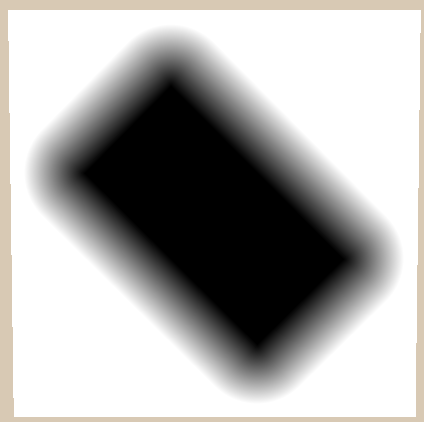While this scales the shape property, it also scales the distance. The main advantage of a signed distance field is that we always know the distance to the nearest surface, but this destroys this property completely. We can fix it easily though by multiplying the distance field we get from the signed distance function (`rectangle` in this case) with the scale. This is also the reason why we can’t easily scale the shape non-uniformly (different scale for x and y axis).

``````float scene(float2 position) {
float2 circlePosition = position;
circlePosition = translate(circlePosition, float2(0, 0));
circlePosition = rotate(circlePosition, .125);
float pulseScale = 1 + 0.5*sin(_Time.y * 3.14);
circlePosition = scale(circlePosition, pulseScale);
float sceneDistance = rectangle(circlePosition, float2(1, 2)) * pulseScale;
return sceneDistance;
}
``````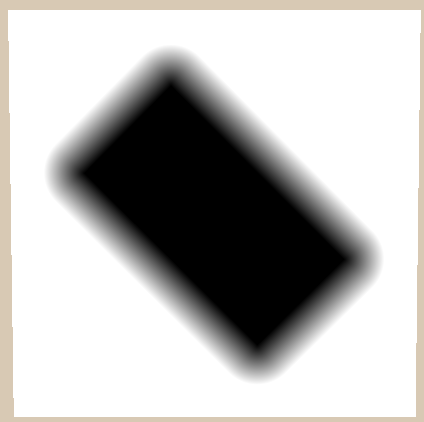## Visualisation

Signed distance fields can be used for a lot of things, for example shadows, 3d scene rendering, physics and text rendering. But we don’t want to get into too complex stuff right now, so I’ll just explain two techniques of visualising them. One a hard shape with antialiasing and the other one renders lines based on the distance.

### Hard Shape

This is a method similar to what’s often used in text rendering and produces a clean shape. If we don’t generate the distance field from a function and instead read it from a texture instead this allows us to use textures with way less resolution than usual and still have a nice result. TextMesh Pro uses this technique for text rendering.

For this technique we use the fact that the data in signed distance fields is continuous and we know the cutoff point. We start by calculating how much the distance field changes to the next pixel. This should be the same amount as the length of the change of the coordinates, but it’s easier and more reliable to evaluate the signed distance.

After we have the change in distance, we can do a smoothstep from half the change in distance to minus plus half the change in distance. That will do a simple cutoff around 0, but with antialiasing. We can then use this antialiased value for whatever we binary value we need. In this example I’m going to change the shader to a transparent shader and use it for the alpha channel. The reason why we do the smoothstep from the positive to the negative value is that we want the negative value of the distance field to be visible. And if you don’t completely understand how the transparent rendering works here, I recommend you read this tutorial I made about transparent rendering.

``````//properties
Properties{
_Color("Color", Color) = (1,1,1,1)
}
``````
``````//in subshader outside of pass
Tags{ "RenderType"="Transparent" "Queue"="Transparent"}

Blend SrcAlpha OneMinusSrcAlpha
ZWrite Off
``````
``````fixed4 frag(v2f i) : SV_TARGET{
float dist = scene(i.worldPos.xz);
float distanceChange = fwidth(dist) * 0.5;
float antialiasedCutoff = smoothstep(distanceChange, -distanceChange, dist);
fixed4 col = fixed4(_Color, antialiasedCutoff);
return col;
}
``````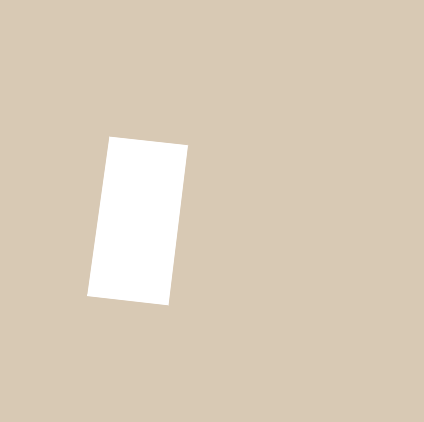### Height Lines

Another common techique of visualising distance fields is to show the distances as lines. In our implementation I’m going to add thick lines and a few smaller ones inbetween. I’m also going to tint the inside and outside of the shape in different colors to make clear where the object is.

We start by showing the difference between the inside and outside of the shape. The colors will be adjustable in the material, so we add new properties as well as shader variables for the inner and outer color of the shape.

``````Properties{
_InsideColor("Inside Color", Color) = (.5, 0, 0, 1)
_OutsideColor("Outside Color", Color) = (0, .5, 0, 1)
}
``````
``````//global shader variables

float4 _InsideColor;
float4 _OutsideColor;
``````

Then in the fragment shader we check whether the pixel we’re rendering is inside or outside the shape by comparing the signed distance to 0 with the `step` function. We use this variable to interpolate from the inner to the outer color and render it to the screen.

``````fixed4 frag(v2f i) : SV_TARGET{
float dist = scene(i.worldPos.xz);
fixed4 col = lerp(_InsideColor, _OutsideColor, step(0, dist));
return col;
}
``````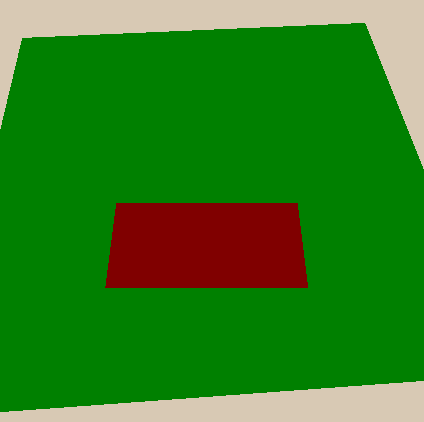To render the lines we first specify how often we render the lines and how thick they are with properties and corresponding shader variables.

``````//Properties
_LineDistance("Mayor Line Distance", Range(0, 2)) = 1
_LineThickness("Mayor Line Thickness", Range(0, 0.1)) = 0.05
``````
``````//shader variables
float _LineDistance;
float _LineThickness;
``````

Then to render the lines, we start by calculating the change in distance to use it for antialiasing later. We also already divide it by 2 because we will add half and subtract half of it later to cover a range of the change of 1 pixel.

``````float distanceChange = fwidth(dist) * 0.5;
``````

Then we take the distance and transform it in a way to make it have similar behaviour at repeating points. To do this we first divide it by the distance between lines, this way we don’t have full numbers every 1 step, instead we have full numbers based on the distance we set.

Then we add 0.5 to the number, take the fractional part and subtract 0.5 again. The fractional part and the subtraction are there to make the line go through zero in a repeating pattern. The reason we add 0.5 before taking the fractional part is to counteract the subtraction of 0.5 later - the offset makes it so the values where our graph is 0 are at 0, 1, 2, etc.. and not 0.5, 1.5, etc… .

The last steps we use to transform the value are to take the absolute value and multiply it by the distance between lines again. The absolute value makes the areas before and after the line points look the same which makes the cutoff for the lines easier to make. The last operation where we multiply the value by the distance between lines again is to counteract the division at the start of the equation, with it the change in the value is the same as at the beginning again and the change in distance we calculated earlier is still valid.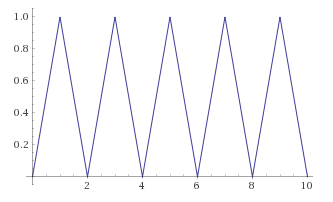``````float majorLineDistance = abs(frac(dist / _LineDistance + 0.5) - 0.5) * _LineDistance;
``````

Now that we calculated the distance to the lines based on the distance to the shape we can draw the lines. We do a smoothstep from the linethickness minus half of the distance change to the linethickness plus half the change in distance and use the line distance we just calculated as the value to compare to. After we have calculated this value we multiply it with the color to make black lines (you could also lerp to another color if you want different colored lines).

``````fixed4 frag(v2f i) : SV_TARGET{
float dist = scene(i.worldPos.xz);
fixed4 col = lerp(_InsideColor, _OutsideColor, step(0, dist));

float distanceChange = fwidth(dist) * 0.5;
float majorLineDistance = abs(frac(dist / _LineDistance + 0.5) - 0.5) * _LineDistance;
float majorLines = smoothstep(_LineThickness - distanceChange, _LineThickness + distanceChange, majorLineDistance);
return col * majorLines;
}
``````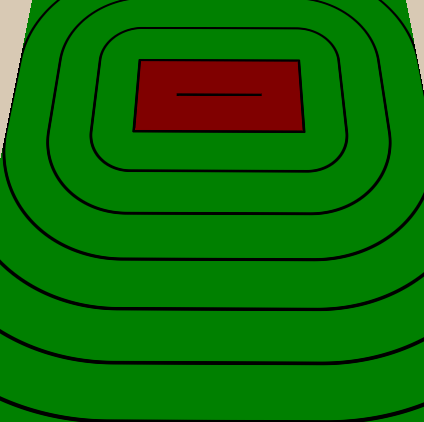The way we implement the sublines between the thick ones is similar, we add a property to specify how many thin lines are inbetween every thick one and then do the same thing we did with the thick lines, but as the distance between thin lines we divide the distance between thick lines with the amount of thin lines inbetween. We’ll also make the thin line amount an `IntRange`, this way we can only assign it whole values and don’t get thin lines that don’t match the thicker ones. After we have calculated the thin lines we multiply them into the color just like the thick ones.

``````//properties
[IntRange]_SubLines("Lines between major lines", Range(1, 10)) = 4
_SubLineThickness("Thickness of inbetween lines", Range(0, 0.05)) = 0.01
``````
``````//shader variables
float _SubLines;
float _SubLineThickness;
``````
``````fixed4 frag(v2f i) : SV_TARGET{
float dist = scene(i.worldPos.xz);
fixed4 col = lerp(_InsideColor, _OutsideColor, step(0, dist));

float distanceChange = fwidth(dist) * 0.5;
float majorLineDistance = abs(frac(dist / _LineDistance + 0.5) - 0.5) * _LineDistance;
float majorLines = smoothstep(_LineThickness - distanceChange, _LineThickness + distanceChange, majorLineDistance);

float distanceBetweenSubLines = _LineDistance / _SubLines;
float subLineDistance = abs(frac(dist / distanceBetweenSubLines + 0.5) - 0.5) * distanceBetweenSubLines;
float subLines = smoothstep(_SubLineThickness - distanceChange, _SubLineThickness + distanceChange, subLineDistance);

return col * majorLines * subLines;
}
``````## Source

### 2d SDF Functions

``````#ifndef SDF_2D
#define SDF_2D

float2 rotate(float2 samplePosition, float rotation){
const float PI = 3.14159;
float angle = rotation * PI * 2 * -1;
float sine, cosine;
sincos(angle, sine, cosine);
return float2(cosine * samplePosition.x + sine * samplePosition.y, cosine * samplePosition.y - sine * samplePosition.x);
}

float2 translate(float2 samplePosition, float2 offset){
//move samplepoint in the opposite direction that we want to move shapes in
return samplePosition - offset;
}

float2 scale(float2 samplePosition, float scale){
return samplePosition / scale;
}

float circle(float2 samplePosition, float radius){
//get distance from center and grow it according to radius
return length(samplePosition) - radius;
}

float rectangle(float2 samplePosition, float2 halfSize){
float2 componentWiseEdgeDistance = abs(samplePosition) - halfSize;
float outsideDistance = length(max(componentWiseEdgeDistance, 0));
float insideDistance = min(max(componentWiseEdgeDistance.x, componentWiseEdgeDistance.y), 0);
return outsideDistance + insideDistance;
}

#endif
``````

### Circle Example

``````Shader "Tutorial/034_2D_SDF_Basics/Circle"{
SubShader{
//the material is completely non-transparent and is rendered at the same time as the other opaque geometry
Tags{ "RenderType"="Opaque" "Queue"="Geometry"}

Pass{
CGPROGRAM

#include "UnityCG.cginc"
#include "2D_SDF.cginc"

#pragma vertex vert
#pragma fragment frag

struct appdata{
float4 vertex : POSITION;
};

struct v2f{
float4 position : SV_POSITION;
float4 worldPos : TEXCOORD0;
};

v2f vert(appdata v){
v2f o;
//calculate the position in clip space to render the object
o.position = UnityObjectToClipPos(v.vertex);
//calculate world position of vertex
o.worldPos = mul(unity_ObjectToWorld, v.vertex);
return o;
}

float scene(float2 position) {
float2 circlePosition = translate(position, float2(3, 2));
float sceneDistance = circle(circlePosition, 2);
return sceneDistance;
}

fixed4 frag(v2f i) : SV_TARGET{
float dist = scene(i.worldPos.xz);
fixed4 col = fixed4(dist, dist, dist, 1);
return col;
}

ENDCG
}
}
FallBack "Standard" //fallback adds a shadow pass so we get shadows on other objects
}
``````

### Rectangle Example

``````Shader "Tutorial/034_2D_SDF_Basics/Rectangle"{

SubShader{
//the material is completely non-transparent and is rendered at the same time as the other opaque geometry
Tags{ "RenderType"="Opaque" "Queue"="Geometry"}

Pass{
CGPROGRAM

#include "UnityCG.cginc"
#include "2D_SDF.cginc"

#pragma vertex vert
#pragma fragment frag

struct appdata{
float4 vertex : POSITION;
};

struct v2f{
float4 position : SV_POSITION;
float4 worldPos : TEXCOORD0;
};

v2f vert(appdata v){
v2f o;
//calculate the position in clip space to render the object
o.position = UnityObjectToClipPos(v.vertex);
//calculate world position of vertex
o.worldPos = mul(unity_ObjectToWorld, v.vertex);
return o;
}

float scene(float2 position) {
float2 circlePosition = position;
circlePosition = rotate(circlePosition, _Time.y * 0.5);
circlePosition = translate(circlePosition, float2(2, 0));
float sceneDistance = rectangle(circlePosition, float2(1, 2));
return sceneDistance;
}

fixed4 frag(v2f i) : SV_TARGET{
float dist = scene(i.worldPos.xz);
fixed4 col = fixed4(dist, dist, dist, 1);
return col;
}

ENDCG
}
}
FallBack "Standard" //fallback adds a shadow pass so we get shadows on other objects
}
``````

### Cutoff

``````Shader "Tutorial/034_2D_SDF_Basics/Cutoff"{
Properties{
_Color("Color", Color) = (1,1,1,1)
}
SubShader{
Tags{ "RenderType"="Transparent" "Queue"="Transparent"}

Blend SrcAlpha OneMinusSrcAlpha
ZWrite Off

Pass{
CGPROGRAM
#include "UnityCG.cginc"
#include "2D_SDF.cginc"

#pragma vertex vert
#pragma fragment frag

struct appdata{
float4 vertex : POSITION;
};

struct v2f{
float4 position : SV_POSITION;
float4 worldPos : TEXCOORD0;
};

fixed3 _Color;

v2f vert(appdata v){
v2f o;
//calculate the position in clip space to render the object
o.position = UnityObjectToClipPos(v.vertex);
//calculate world position of vertex
o.worldPos = mul(unity_ObjectToWorld, v.vertex);
return o;
}

float scene(float2 position) {
float2 circlePosition = position;
circlePosition = rotate(circlePosition, _Time.y * 0.5);
circlePosition = translate(circlePosition, float2(2, 0));
float sceneDistance = rectangle(circlePosition, float2(1, 2));
return sceneDistance;
}

fixed4 frag(v2f i) : SV_TARGET{
float dist = scene(i.worldPos.xz);
float distanceChange = fwidth(dist) * 0.5;
float antialiasedCutoff = smoothstep(distanceChange, -distanceChange, dist);
fixed4 col = fixed4(_Color, antialiasedCutoff);
return col;
}

ENDCG
}
}
FallBack "Standard" //fallback adds a shadow pass so we get shadows on other objects
}
``````

### Distance Lines

``````Shader "Tutorial/034_2D_SDF_Basics/DistanceLines"{
Properties{
_InsideColor("Inside Color", Color) = (.5, 0, 0, 1)
_OutsideColor("Outside Color", Color) = (0, .5, 0, 1)

_LineDistance("Mayor Line Distance", Range(0, 2)) = 1
_LineThickness("Mayor Line Thickness", Range(0, 0.1)) = 0.05

[IntRange]_SubLines("Lines between major lines", Range(1, 10)) = 4
_SubLineThickness("Thickness of inbetween lines", Range(0, 0.05)) = 0.01
}

SubShader{
//the material is completely non-transparent and is rendered at the same time as the other opaque geometry
Tags{ "RenderType"="Opaque" "Queue"="Geometry"}

Pass{
CGPROGRAM

#include "UnityCG.cginc"
#include "2D_SDF.cginc"

#pragma vertex vert
#pragma fragment frag

struct appdata{
float4 vertex : POSITION;
};

struct v2f{
float4 position : SV_POSITION;
float4 worldPos : TEXCOORD0;
};

v2f vert(appdata v){
v2f o;
//calculate the position in clip space to render the object
o.position = UnityObjectToClipPos(v.vertex);
//calculate world position of vertex
o.worldPos = mul(unity_ObjectToWorld, v.vertex);
return o;
}

float scene(float2 position) {
float2 circlePosition = position;
circlePosition = rotate(circlePosition, _Time.y * 0.2);
circlePosition = translate(circlePosition, float2(2, 0));
float sceneDistance = rectangle(circlePosition, float2(1, 2));
return sceneDistance;
}

float4 _InsideColor;
float4 _OutsideColor;

float _LineDistance;
float _LineThickness;

float _SubLines;
float _SubLineThickness;

fixed4 frag(v2f i) : SV_TARGET{
float dist = scene(i.worldPos.xz);
fixed4 col = lerp(_InsideColor, _OutsideColor, step(0, dist));

float distanceChange = fwidth(dist) * 0.5;
float majorLineDistance = abs(frac(dist / _LineDistance + 0.5) - 0.5) * _LineDistance;
float majorLines = smoothstep(_LineThickness - distanceChange, _LineThickness + distanceChange, majorLineDistance);

float distanceBetweenSubLines = _LineDistance / _SubLines;
float subLineDistance = abs(frac(dist / distanceBetweenSubLines + 0.5) - 0.5) * distanceBetweenSubLines;
float subLines = smoothstep(_SubLineThickness - distanceChange, _SubLineThickness + distanceChange, subLineDistance);

return col * majorLines * subLines;
}

ENDCG
}
}
FallBack "Standard" //fallback adds a shadow pass so we get shadows on other objects
}
``````

I hope I was able to explain the basics of signed distance fields to you and that you’re exited for the next few tutorials where I explain more ways to do things with them.

You can also find me on twitter at @totallyRonja. If you liked my tutorial and want to support me you can do that on Patreon (patreon.com/RonjaTutorials) or Ko-Fi (ko-fi.com/RonjaTutorials).